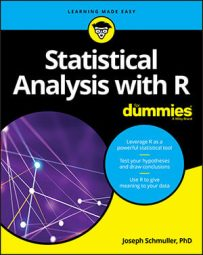##### Statistical Analysis with R For DummiesAn R function called `z.test() `would be great for doing the kind of testing in which you use z-scores in the hypothesis test. One problem: That function does not exist in base R. Although you can find one in other packages, it's easy enough to create one and learn a bit about R programming in the process.

The function will work like this:

`> IQ.data <- c(100,101,104,109,125,116,105,108,110)`

```> z.test(IQ.data,100,15) ``` ```z = 1.733 ``` ```one-tailed probability = 0.042 ``` ```two-tailed probability = 0.084 ``` Begin by creating the function name and its arguments:

```z.test = function(x,mu,popvar){ ``` The first argument is the vector of data, the second is the population mean, and the third is the population variance. The left curly bracket signifies that the remainder of the code is what happens inside the function.

Next, create a vector that will hold the one-tailed probability of the z-score you'll calculate:

```one.tail.p <- NULL ``` Then you calculate the z-score and round it to three decimal places:

```z.score <- round((mean(x)-mu)/(popvar/sqrt(length(x))),3) ``` Without the rounding, R might calculate many decimal places, and the output would look messy.

Finally, you calculate the one-tailed probability (the proportion of area beyond the calculated z-score), and again round to three decimal places:

```one.tail.p <- round(pnorm(abs(z.score),lower.tail = FALSE),3) ``` Why put `abs() `(absolute value) in the argument to `pnorm`? Remember that an alternative hypothesis can specify a value below the mean, and the data might result in a negative z-score.

The next order of business is to set up the output display. For this, you use the `cat() `function. The name cat is short for concatenate and print, which is exactly what you want you to do here: Concatenate (put together) strings (like `one-tailed probability =`) with expressions (like `one.tail.p`), and then show that whole thing onscreen. You also want you to start a new line for each concatenation, and `\n` is R's way of making that happen.

Here's the cat statement:

```cat(" z =",z.score,"\n", ``````"one-tailed probability =", one.tail.p,"\n", ``` ```"two-tailed probability =", 2*one.tail.p )} ``` The space between the left quote and `z` lines up the first line with the next two onscreen. The right curly bracket closes off the function.

Here it is, all together:

```z.test = function(x,mu,popvar){ ``` `one.tail.p <- NULL`

```z.score <- round((mean(x)-mu)/(popvar/sqrt(length(x))),3) ``` ```one.tail.p <- round(pnorm(abs(z.score),lower.tail = FALSE),3) ``` `cat(" z =",z.score,"\n",`

`"one-tailed probability =", one.tail.p,"\n",`

`"two-tailed probability =", 2*one.tail.p )}`